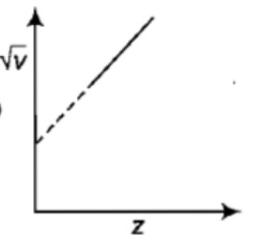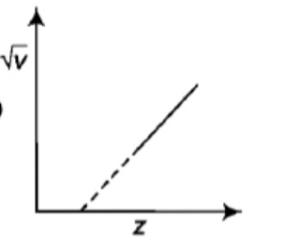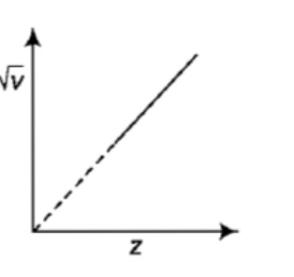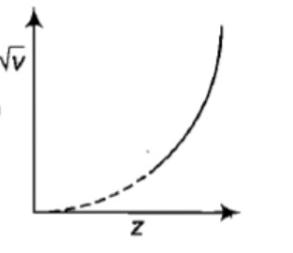The photon radiated from hydrogen corresponding to the line of Lyman series is absorbed by a hydrogen-like atom X in the excited state.   As a result the hydrogen-like atom X makes a transition to ${n}^{th}$ orbit. Then:

(1) X =                 (2) X = $L{i}^{++}$, n = 6

(3) X =                  (4) X = $L{i}^{++}$, n = 9

Concept Questions :-

Spectral series
High Yielding Test Series + Question Bank - NEET 2020

Difficulty Level:

If an electron in an hydrogen atom jumps from an orbit ${n}_{i}=3$ to an orbit with level ${n}_{f}=2$, the frequency of the emitted radiation is

(1)                (2) v = $\frac{CR}{6}$                (3) v =              (4) v  =

Concept Questions :-

Bohr's model of atom
High Yielding Test Series + Question Bank - NEET 2020

Difficulty Level:

The energy of a hydrogen atom in its ground state is -13.6 eV. The energy of the level corresponding to the quantum number n = 5 is

(1) - 0.54 eV          (2) - 5.40 eV          (3) - 0.85 eV          (4) -2.72 eV

Concept Questions :-

Bohr's model of atom
High Yielding Test Series + Question Bank - NEET 2020

Difficulty Level:

The wave number of a photon in Brackett series of hydrogen atom is $\frac{9}{400}R$. The electron has transited from the orbit havit quantum number [Odisha JEE 2012]

(1) 5              (2) 6             (3) 4                 (4) 7

Concept Questions :-

Spectral series
High Yielding Test Series + Question Bank - NEET 2020

Difficulty Level:

In an experiment to determine the e/m value for an electron using Thomson's method the electrostatic deflection plates were 0.01 m apart and had a potential difference of 200 volts applied. Then the electric field strength between the plates is

(1)                           (2)

(3)                           (4)

Concept Questions :-

Various atomic model
High Yielding Test Series + Question Bank - NEET 2020

Difficulty Level:

Which of the following spectral series in hydrogen atom gives spectral line of 4860 $\stackrel{0}{A}$?

(1) Lyman          (2) Balmer         (3) Paschen             (4) Brackett

Concept Questions :-

Spectral series
High Yielding Test Series + Question Bank - NEET 2020

Difficulty Level:

Hydrogen $\left({}_{1}H_{1}\right)$, Deuterium $\left({}_{1}H_{2}\right)$, singly ionised Helium ${\left({}_{2}H{e}^{4}\right)}^{+}$ and doubly ionised lithium ${\left({}_{3}L{i}^{6}\right)}^{++}$ all have one electron around  the nucleus. Consider an electron transition from n = 2 to n = 1. If the wave lengths of emitted radiation are  and ${\lambda }_{4}$ respectively then approximately which one of the following is correct [JEE (Main) 2014]

(1) $4{\lambda }_{1}=2{\lambda }_{2}=2{\lambda }_{3}={\lambda }_{4}$                  (2) ${\lambda }_{1}=2{\lambda }_{2}=2{\lambda }_{3}={\lambda }_{4}$

(3) ${\lambda }_{1}={\lambda }_{2}=4{\lambda }_{3}=9{\lambda }_{4}$                    (4) ${\lambda }_{1}=2{\lambda }_{2}=3{\lambda }_{3}={\lambda }_{4}$

Concept Questions :-

Bohr's model of atom
High Yielding Test Series + Question Bank - NEET 2020

Difficulty Level:

To explain his theory, Bohr used

(1) conservation of linear momentum

(2) conservation of angular momentum

(3) conservation of quantum  frequency

(4) conservation of energy

Concept Questions :-

Bohr's model of atom
High Yielding Test Series + Question Bank - NEET 2020

Difficulty Level:

The minimum wavelength of X-rays produced by electrons accelerated by a potential difference of V volt is equal to

(1) $\frac{eV}{hc}$               (2) $\frac{eh}{cV}$               (3) $\frac{hc}{eV}$               (4) $\frac{cV}{eh}$

Concept Questions :-

X-ray
High Yielding Test Series + Question Bank - NEET 2020

Difficulty Level:

The graph between the square root of the frequency of a specific line of the characteristic spectrum of X-rays and the atomic number of the target will be:

(1)(2)(3)(4)Concept Questions :-

X-ray